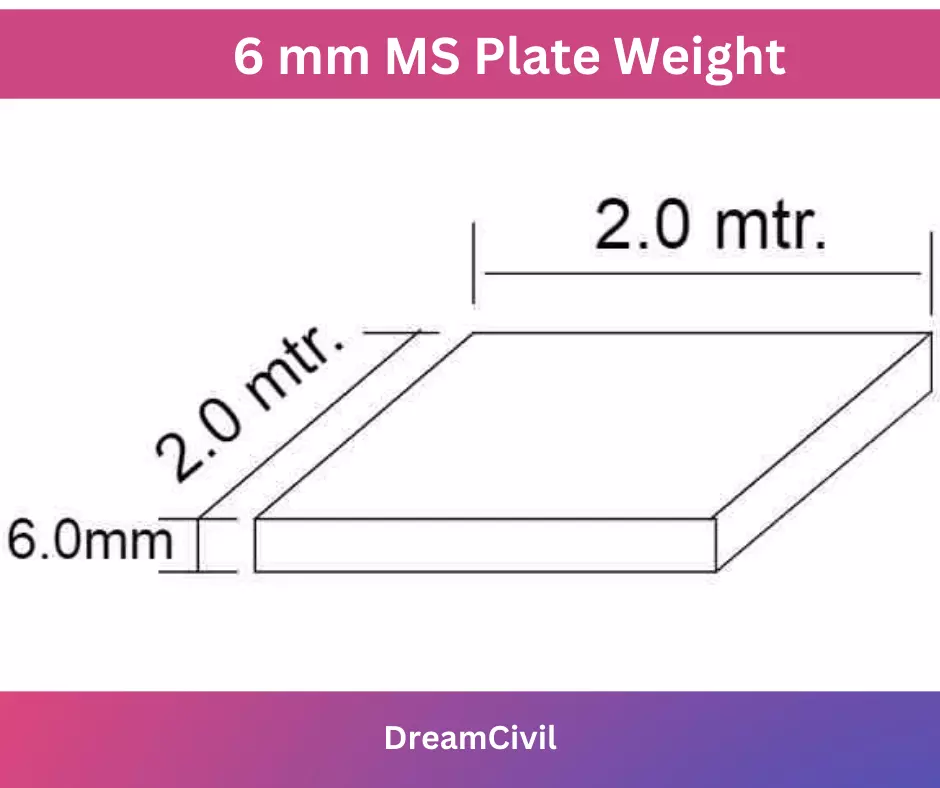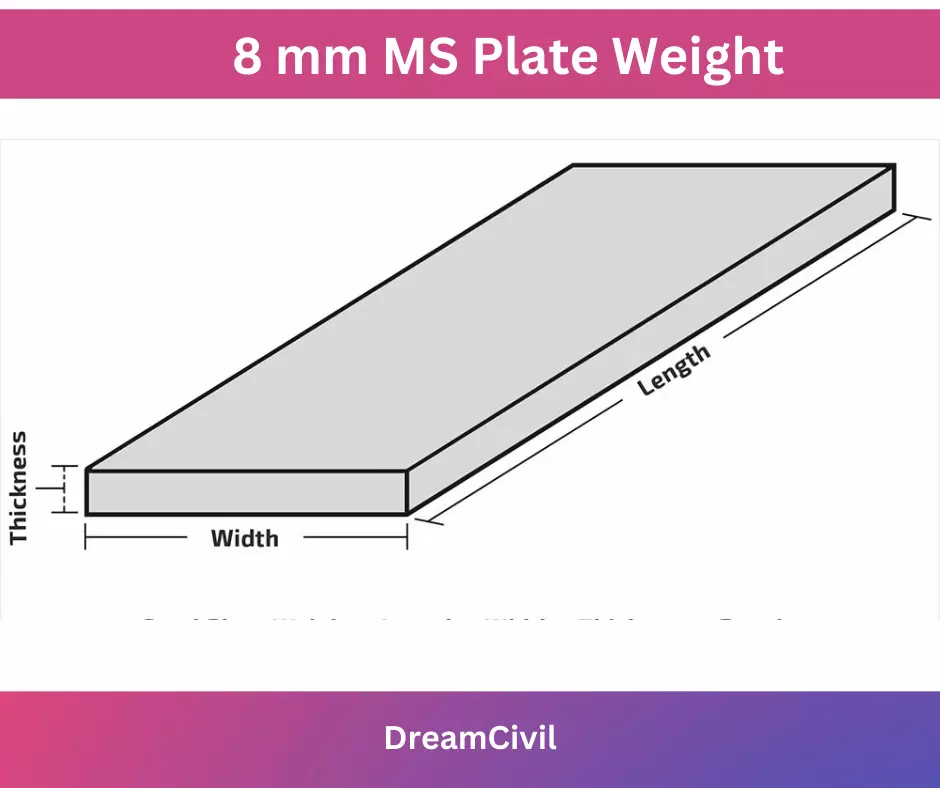Monday, October 2, 2023

# Unit Weight of the Ms Plate: 6,8,10,12.5,12,20 mm Ms Plate Weight

The unit weight of the ms plate is measured per square foot and per square meter, this is calculated as the unit weight of ms plates. As we already know Ms plates have various sizes like 6 mm,8 mm,10 mm,12 mm, and 20 mm thick. We weigh the ms plate formula for the calculation.

### A. Unit Weight of the Ms Plate

The weight of Ms plates is the multiplication of volume and density. The density of steel is 7850 Kg/m3 already known.

The weight of the Ms plate formula.

Weight = volume × density

Weight of MS Plate = Volume × density

For Example:

When the Length of the Ms plate is 1m, the breadth of the Ms plate is 1m, and also a thickness of 1m then,

Weight of MS Plate = Volume × density

=  1 x 7850

Weight of Ms Plate= 7850 Kg/m2

#### 1. 6 mm MS plate weightGiven,

Thickness of ms plate = 6 mm = 0.006 m

Length of ms plate = 1m

Width of ms plate = 1m

Area of ms plate = 1×1 m2 = 1 m2

Now,

volume = Area × thickness

Volume = 1m2 ×0.006 m = 0.006 m3

density of steel = 7850 Kg/m3

Using the formula,

Weight of ms plate 6 mm = volume ×density

weight of ms plate 6 mm = 0.006 m3×7850 Kg/m3

6 mm ms plate weight = 47.1 Kg/m2

That is 6mm ms plate weight per sq. meter

Now,

calculate the weight of the ms plate 6 mm per sq. feet

We know that 1m2 = 10.764 sq.ft

So the weight of ms plate 6 mm per sq. feet is

= 47.1/10.764

= 4.376 Kg/ft2

#### 2.8 mm thick ms plate weightWe have given thickness of ms plate = 8 mm = 0.008 m

Length of ms plate = 1m

Width of ms plate = 1m

Area of ms plate = 1×1 m2 = 1 m2

Now,

Volume = Area × thickness

Volume = 1m2 ×0.008 m = 0.008 m3

Density of steel = 7850 Kg/m3

8 mm thick ms plate weight = Volume × Density

Weight of 8 mm ms plate = 0.008 m3×7850 Kg/m3

8 mm thick ms plate weight = 62.8 Kg/m2

That is the weight of the MS plate is 8mm per sq. meter

Now,

Calculate the weight of the ms plate 8mm per sq. feet

We know that 1m2 = 10.764 sq.ft

The weight of the ms plate 8mm per sq. foot is

= 62.8/10.764

= 5.834 Kg/ft2

#### 3. 10 mm MS Plate Weight

We have given thickness of ms plate = 10mm = 0.010 m

Length of ms plate = 1m

Width of ms plate = 1m

Area of ms plate = 1×1 m2 = 1 m2

Now,

Volume = Area × thickness

Volume = 1m2 ×0.010 m = 0.010 m3

Density of steel = 7850 Kg/m3

Now,

10 mm ms plate weight = Volume × Density

weight of ms plate 10mm = 0.010 m3 × 7850 Kg/m3

weight of ms plate 10mm = 78.5 Kg/m2

That is 10 mm ms plate weight per square meter

Now,

Calculate the weight of the ms plate 10mm per sq. feet

We know that 1m2 = 10.764 sq.ft

The weight of the ms plate 10mm per sq. feet is

= 78.5/10.764

= 7.29 Kg/ft2

#### 4. Weight of ms plate 12.5mm

We have given thickness of ms plate = 12.5mm = 0.0125 m

Length of ms plate = 1m

Width of ms plate = 1m

Area of ms plate = 1×1 m2 = 1 m2

Now volume = Area × thickness

Volume = 1m2 ×0.0125 m = 0.0125 m3

Density of steel = 7850 Kg/m3

Now,

Weight of ms plate 12.5mm = Volume × Density

weight of ms plate 12.5mm = 0.0125 m3 ×7850 Kg/m3

Weight of ms plate 12.5mm = 98.1 Kg/m2

That is the weight of the ms plate is 12.5mm per sq. meter

Now,

Calculate the weight of the ms plate 12.5mm per sq. feet

We know that 1m2 = 10.764 sq.ft

So the weight of ms plate 12.5mm per sq. feet is

= 98.1/10.764

= 9.113 Kg/ft2

#### 5. 12 mm thick ms plate weight

We have given thickness of ms plate = 12 mm = 0.0120 m

Length of ms plate = 1m

Width of ms plate = 1m

Area of ms plate = 1×1 m2 = 1 m2

Now,

Volume = Area × thickness

Volume = 1m2 ×0.0120 m = 0.0120 m3

Density of steel = 7850 Kg/m3

Now, 12 mm thick ms plate weight = volume × density

weight of ms plate 12 mm = 0.0120 m3×7850 Kg/m3

12 mm thick ms plate weight = 94.2 Kg/m2

That is the weight of the MS plate is 12.5 mm per sq. meter

Now calculate the weight of the ms plate 12 mm per sq. feet

We know that 1m2 = 10.764 sq.ft

So the weight of ms plate 12 mm per sq. feet is

= 94.2/10.764

= 8.75 Kg/ft2

#### 6. 20 mm thick ms plate weight

We have given thickness of ms plate = 12 mm = 0.0120 m

Length of ms plate = 1m

Width of ms plate = 1m

Area of ms plate = 1×1 m2 = 1 m2

Now volume = Area × thickness

Volume = 1m2 ×0.0120 m = 0.0120 m3

density of steel = 7850 Kg/m3

Now 20 mm thick ms plate weight = Volume × Density

Weight of ms plate 20 mm = 0.0200 m3×7850 Kg/m3

20 mm thick ms plate weight = 157 Kg/m2

That is the weight of the ms plate is 20mm per sq. meter

Now,

Calculate the weight of the ms plate 20 mm per sq. feet

We know that 1m2 = 10.764 sq.ft

The weight of the ms plate 20 mm per sq. foot is

= 157/10.764

= 14.585 Kg/ft2

Hope you know the unit weight of the ms Plate

 Read Also: Safety Tips for Dam ConstructionHe is a founder and lead author of Dream Civil International and his civil engineering research articles has been taken as source by world's top news and educational sites like USA Today, Time, The richest, Wikipedia, etc.
Latest Articles

Related Articles